# Static Variable in Java

Challenge Inside! : Find out where you stand! Try quiz, solve problems & win rewards!
Learn via video courseJava Course - Mastering the Fundamentals
By Tarun LuthraFree
5Enrolled: 1000Java Course - Mastering the Fundamentals
Tarun LuthraFree
5
Enrolled: 1000

## Overview

A static variable is associated with a class rather than an instance. A static field is declared using the static keyword. Static variables are shared across all instances of a class.

There is only one copy of a static variable per class. Non-static variables cannot be called inside static methods.

If any instance of a class modifies the value of a static variable, the change is reflected across all instances of the class. static variable in java are memory-efficient as they are not duplicated for each instance.

## What is a Static Variable in Java?

Let’s start this article with a real life example. Consider a machine that produces different varieties of pens like blue, black, green, etc. for a company ‘X’.

All pens are different in their properties like some of them are blue having different body structure and some of them are black. But one attribute is common among all the pens is its company name i.e ‘X’.

Let's implement a class Pen incorporating the details we mentioned above.

The companyName variable is of static type which implies it is shared among all instances of the Pen class.

What is the purpose of declaring the companyName variable as static? It is because since the value of companyName variable is same for all the Pen objects, maintaining it separately for each instance is not memory efficient. Hence, the static variable is associated with the class rather than objects.

## Static Variable in Java

• The static variables are those variables that are common to all the instances of the class.
• Only a single copy of the static variable is created and shared among all the instances of the class.
• Because it is a class-level variable, memory allocation of such variables only happens once when the class is loaded in the memory.
• If an object modifies the value of a static variable, the change is reflected across all objects.

## What is the Use of static Keyword in Java

The main use of the static keyword in java is used for memory management in java. Whenever we place a static keyword before the initialization of a particular class’s methods or variables, these static methods and variables belong to the class instead of their instances or objects.

### Syntax of Static Variable in Java

Syntax: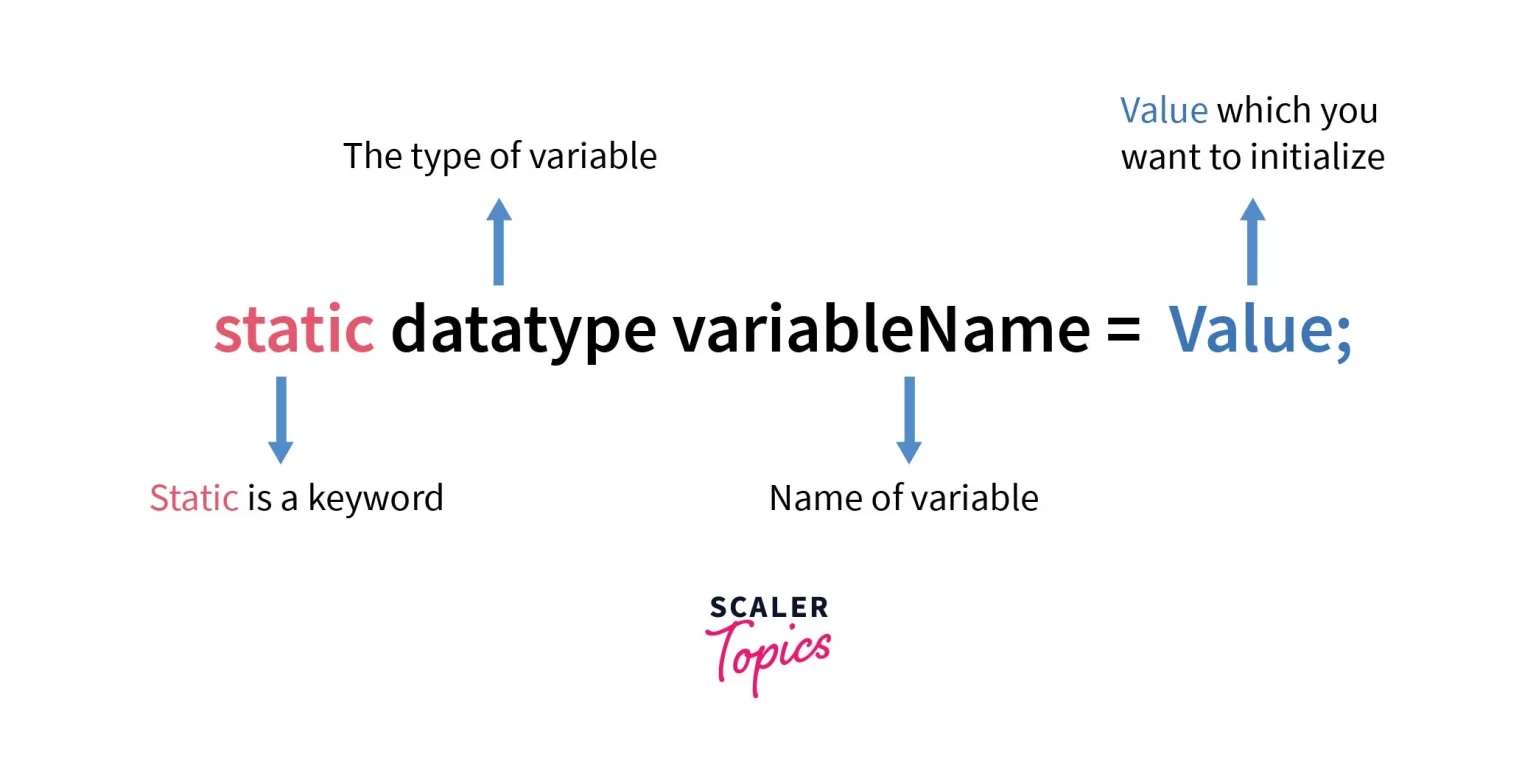Example: Country.java

Output:

Explanation:

• In the example above, we have created a class Country with a static variable countryCounter.
• We have created two objects: ob1 and ob2 of Country class and incremented the value of countryCounter using them.
• Finally, we have accessed the value of countryCounter variable in three different ways and the value is the same as it can be seen in the output.
• This shows the variable is shared across all instances and it can be accessed using the class identifier as well.

Working on the above program diagrammatically.

During compilation of the Country.java file, the java compiler binds the static variables with the class as in this case countryCounter variable is bind to the compiled class.

We can see in the below diagram that the static variable countryCounter is linked to the class and this variable is shared among all the class instances.

In this case, two instances of the country class are accessing the same static variable shown below in the diagram. Since the countryCounter variable is a static variable and static variable is a class variable so any changes in these variables are reflected among all the instances of the class so after the first increment the value of countryCounter is 1 and after another increment, the final value is 2.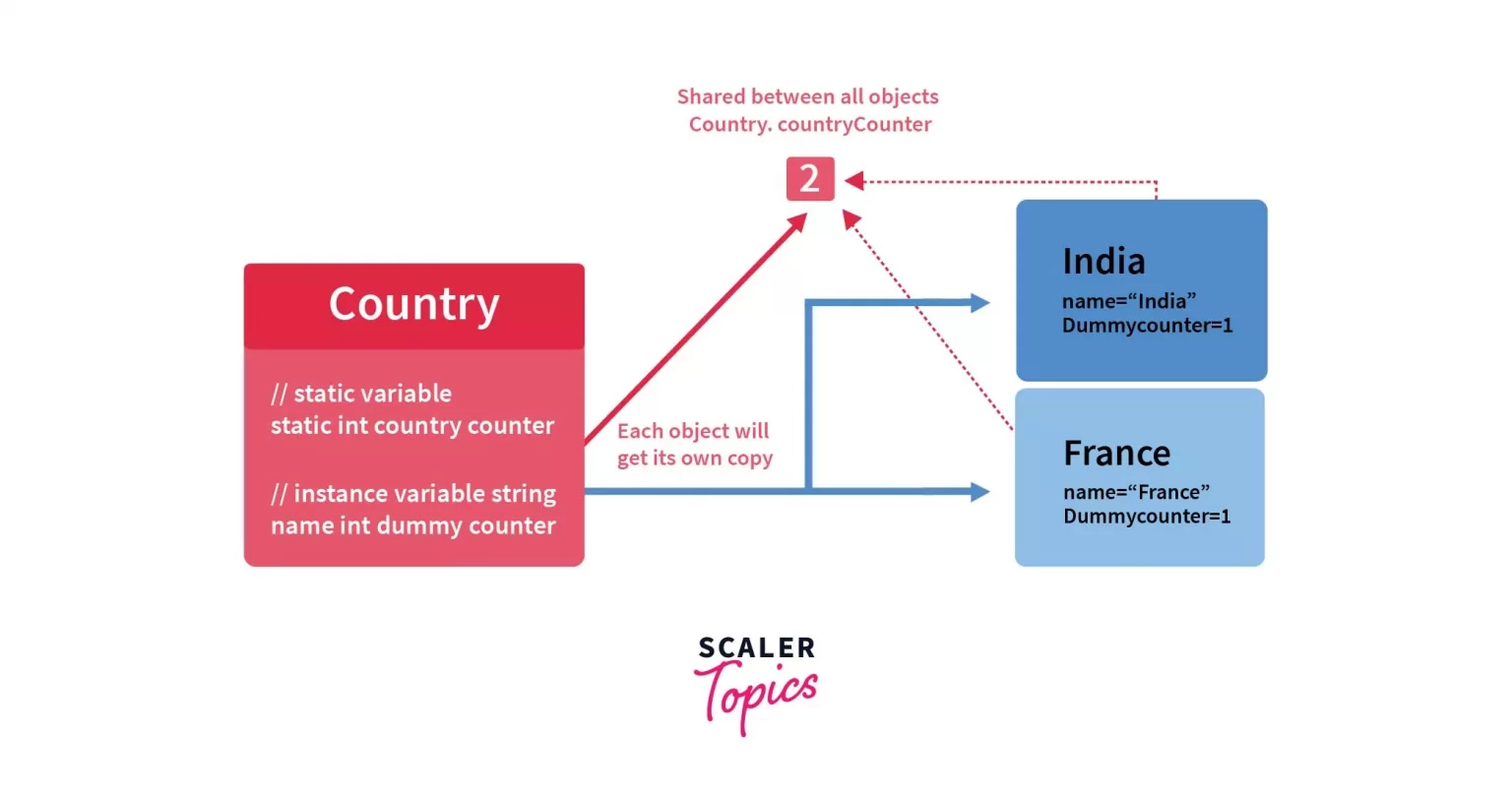## Storage Area of Static Variable in Java

The static variables and methods are stored in the heap memory. In fact all static methods are stored in the Heap memory.

Before the Java 8 version, static variables of the class were stored in the separate section of the non-heap memory named as Method Area created by the Java Virtual Machine after the class compilation. Method area section was used to store static variables of the class, metadata of the class, etc. Whereas, non-static methods and variables were stored in the heap memory.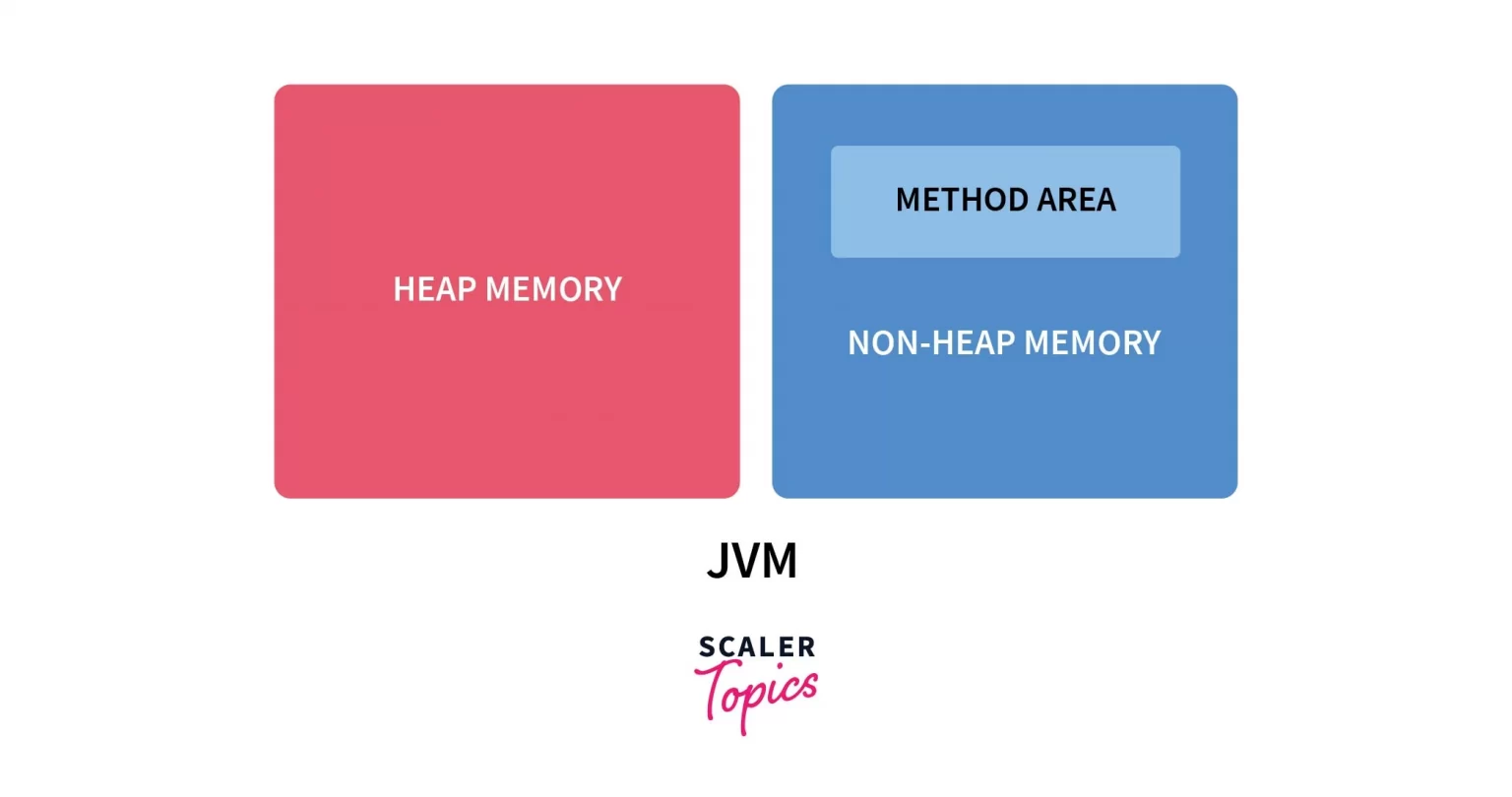## How to Declare a Static Variable in Java

static variable in java are declared through the use of static keyword. The static keyword indicates to the JVM (Java Virtual Machine) that this variable should be loaded with the class during the compilation of the program.

If we don’t initialize the static variable the default value of the static variable data type will be automatically assigned to the static variable in java. For example, the default value of integers is zero, objects is null and so on.

### Declaration Examples

Example:

Output:

Explanation:

• If we don’t assign an initial value to any static variable the default value is automatically initialized to that particular variable.
• In the above example, there are static variables one of boolean type and the second is an integer type.
• We didn’t initialize these variables so whenever we try to access these variables we will get the default values of these variables: false for boolean type variable and the 0 for integer. These are the default values for these data types.

## Declaration Scope of the Static Variable in Java

We cannot declare static variables in the main() method or any kind of method of the class. The static variables must be declared as a class member in the class.

Because during compilation time JVM binds static variables to the class level that means they have to be declared as class members.

Example

Explanation:

• The static variable in java can be declared like class members of the class like static int number, is the valid declaration of the static variable but static variables cannot be declared inside any method scope.
• If we try to declare static variables inside any method, the compiler will show a syntax error.
• In the above example if we try to declare a static variable in the main() method the compiler will show an illegal modifier syntax error.

## Initialization of Static Final Variables in Java

The static variables are also used as constants with the final keyword in Java. Constants must be initialized and any kind of update is not allowed on them. Let’s understand this with an example.

• Variables declared with static and final keywords must be initialized with a value.
• Final variables cannot be modified as shown below.
• In the above example, if we don’t initialize the static variables compiler will show an error during compilation time.
• In the above program final static variable b is not initialized and hence it will throw an compile-time error.
• The final keyword restricts any changes in the variable so any kind of increment, decrement, or any other change will violate this restriction and that’s why the compiler will show an error in the above case “a++” line is not a valid statement.

## Accessibility of the Static Variable in Java

Static variables can be accessed by calling the class name of the class. There is no need to create an instance of the class for accessing the static variables because static variables are the class variables and are shared among all the class instances.

• The static variables can be accessed in all types of methods: static or non-static.
• We cannot access non-static variables in static methods because non-static variables can only be accessed by creating an instance of the class.
• However, static methods can be called without creating an instance of the class.
• This leads to reference error for the non-static variable because non-static variables can be accessed only through an instance of the class that’s why static methods cannot access non-static variables.
• Another way of understanding this: If we have not created any instance of a class and call the static method which contains a non-static reference, which object should the non-static member point to?

Code:

Explanation:

• In the above example, a non-static variable named number is called in two methods: one is a static method check() and the other is a non-static method i.e check1().
• When the non-static variable is called inside check(), Java compiler will show a reference error because:
• We can only access the non-static variable only after creating an instance of the class.
• But static methods can be called without creating an instance of the class,
• That’s why during accessing the non-static variable in the static method compiler will show reference error.

### Syntax for Accessing

• Classname: Name of the class containing the static variable.
• (.) dot operator: Operator used to access static variables.
• Variablename: Name of static variable that needs to be called.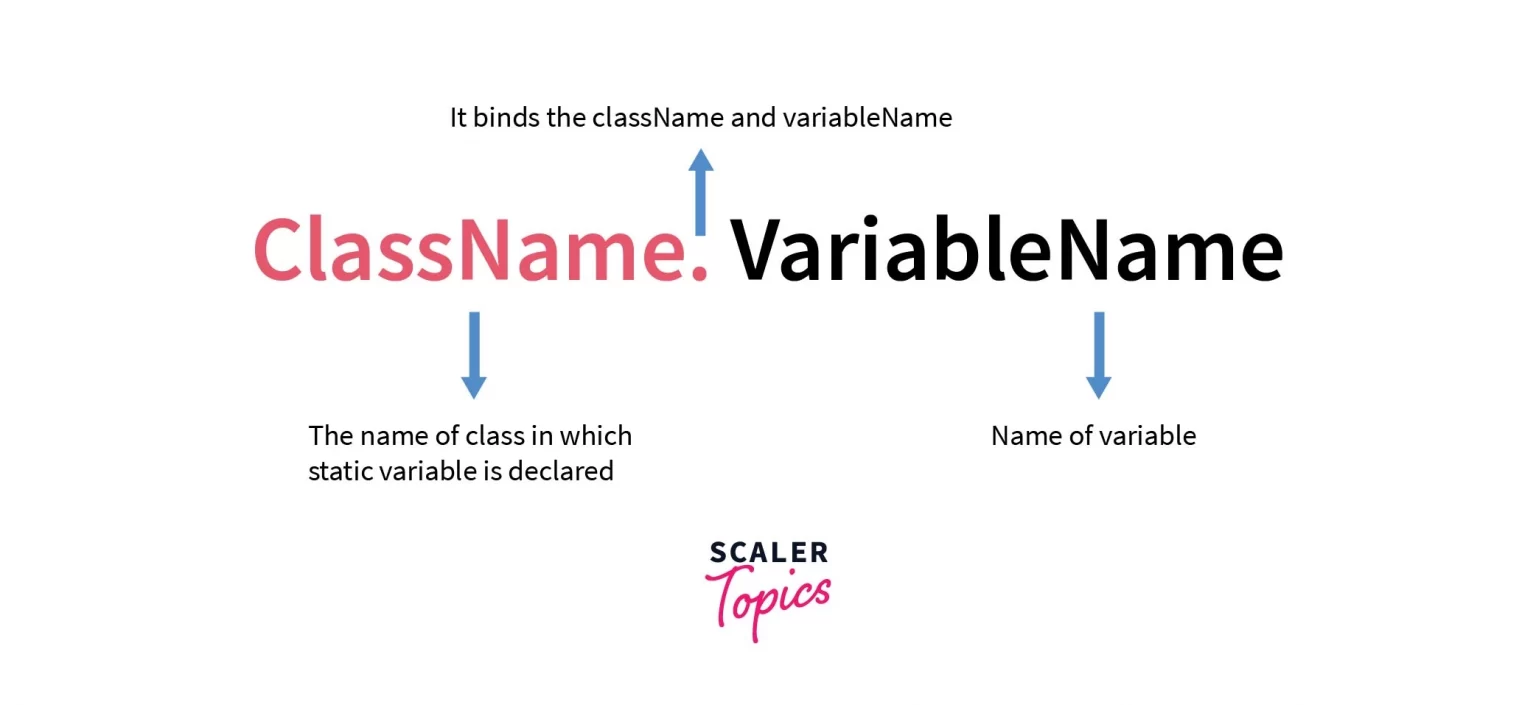Example

Output:

Explanation:

• In the above example, the static variable named ans is accessed in two ways. One way is without creating an instance of the class using the class reference only and the second way is using the instance of the class.
• While accessing the static variable in java using the instance of the class, the compiler will show a warning: The static field Main.ans should be accessed in a static way.
• This is because there is no need to create an instance of accessing the static members of the class.

### 1. Common for all instances

The static variables are common for all the instances. A single copy of the static variables is shared among all the instances of the class. If any object of the class modifies the value of a static field, the modification is reflected across all instances.

Example

Output:

Explanation:

• In the above example, a single copy of the static variable in java, i.e, number is shared among all the two instances of the class.
• First instance named as ob1 increments the value of number variable by 1 and the second instance named as ob2 again increments the value of a static variable number by 1 finally making it 2,

### Class containing static members and non-static members

Code:

Output:

• In the above program, static variable insert() is called by every new instance of the class and it inserts the name for every new instance and increments the count_clicks variable.
• Since a static variable is shared among all the instances, any changes done due to any instance automatically update its original state.
• In the above program, we increment static members three times. Hence the output is 3. This value is same across all instances, which means changes done by any one instance is reflected across all instances. Use this Compiler to compile your Java code.

### Memory Management of the above program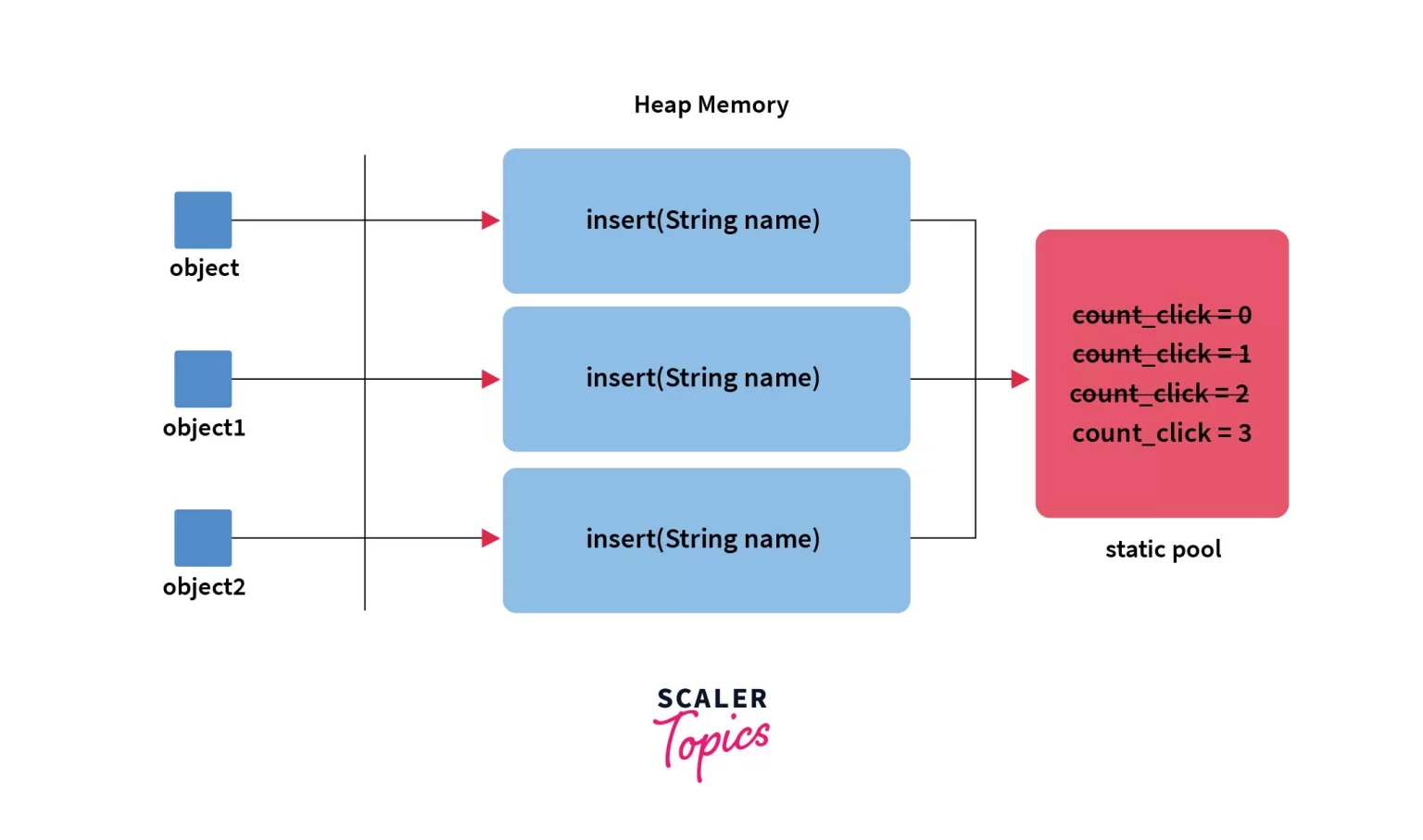• Whenever we create an instance of the class, a separate copy of all the class methods and variables except static members is created for all respective instances of the class in the heap memory.
• In the above example the method name as insert() is called by the three instances of the class and each time insert() method is called the value of count_clicks is increment by one.
• Since count_clicks is a static variable, it is shared among all the instances of the class so any changes on this variable are reflected among all the instances of the class.

## A Real-Life Example of Static Variable in Java

• The simplest real-life example of a static variable is supposed to have a class named as Student that is used to represent the details of any student that took admission in the school.
• Now we have to count how many students took admission in the school.
• In this case, the concept of static variable in java can be used. We simply create a static variable named count_student inside the Student class.
• Increment the value of count_student inside the constructor for Student class. As a result, whenever we create a new instance of Student class, count_student will automatically increase by 1.
• In this way we can count the total number of new students took admission in the school.

## Conclusion

• Static variable in Java are shared across all instances of a class. Static variables are associated with the class rather than with the objects.
• Static variables are loaded at the time of class compilation.
• Static variables can be used in any type of methods: static or non-static.
• Non-static variables cannot be used inside static methods. It will throw a compile-time error.
• Static variables are memory efficient as they are created only once per class. They are not created separately for each instance like instance variables.
• Static variables can be accesed using either the class name or instance name. However, it is recommend to access static variables using the class name.